# C++语言篇 第九章 自定义函数详解

## 序言

C++ 中的函数由一段相对独立的代码组成，这段代码能实现某一项具体、独立、完整的功能。

## 一、使用函数的目的：

①“代码重用”：代码重用是保证同一个函数可以被一个或多个函数调用任意多次，从而减少重复代码的编写。

②“问题分解”：问题分解可以保证一个大的程序（或者说软件），按照模块化编程思想，由大化小，分解成若干个结构清晰、功能独立、调试方便的函数，甚至给若干人合作完成，从而提高开发效率。

## 二、函数有两种：

二种是自定义函数:用户自已根据需要编写的函数。

## 三、自定义函数的定义: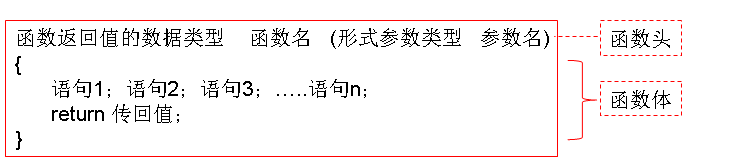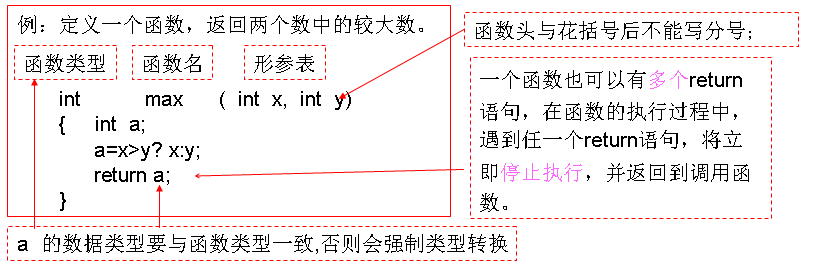## 四、返回值

１、如果在定义函数时不指定函数类型，系统会隐含指定函数类型为int，函数结束也需返回一个int型值。

２、函数的返回值由 return 语句给出。 return（表达式）  或 return  表达式；

３、如果函数没有返回值，函数名前的类型标识符为void，return 语句可省略不写。

４、如果return中的值与函数值的类型不一致，则以函数类型为准。即在返回时先作隐含的类型转换，然后再返回。如果类型不相容，不能转换，则编译会出错

#include<bits/stdc++.h>
using namespace std;

int  f(void)
{ return 3.5;}  //可以写成return 3.5也可以写成 return (3.5);

int main()
{   int a;
a=f();
cout<<“a=”<<a;
}


3.5 被转换成3后，返回给f()函数。 输出a=3。

#include<bits/stdc++.h>
using namespace std;
int main()
{ float a,b;
int c;
int max(float x,float y);
scanf("%f %f",&a,&b);
c=max(a,b);
printf("Max is %d\n",c);
}
int max(float x, float y)
{ if(x>y) return x;
else    return y;
}


## 五、函数的四种类型

### 1、有参数无返回值

#include<bits/stdc++.h>
using namespace std;

void fun(int   n)  //无返回值函数要写void类型
{ int i;
for(i=1; i<=n; i++)
cout<<i;
cout<<endl;
return;　　　    //无返回值函数可以写return；也可以不写
}
int main()
{  int x;
cin>>x;
fun(x);
return 0;
} 

### 2、有参数有返回值

#include<bits/stdc++.h>
using namespace std;

long power(int x, int n)
{     long  p=1;
for(int i=1; i<=n; i++)     p=p*x;
return p;     //可以写成return p；也可以写成return (p);
}

int main()
{  int a,b,c;
cin>>a>>b;
c=power(a,b);
cout<<c;
}


### 3、无参数无返回值

#include<bits/stdc++.h>
using namespace std;

void show1(void)
{   cout<<"***";}

int main()
{  show1();
return;    //无返回值函数也可以用return; 但后面不接变量，也不会返回值。
}


### ４、无参数有返回值

#include<bits/stdc++.h>
using namespace std;

int geti(void)
{   int i; cin>>i;
return i;
}

int main()
{  int a; a=geti(); cout<<a;}


## 六、函数的声明：

### 函数原型的通式为：

#include<bits/stdc++.h>
using namespace std;

int js(int n) 　　　　//js()函数定义在主函数前，所以不要声明

{  int z,i;
z=1;
for (i=1;i<=n;i++)
z=z * i;
return z;
}

int main()
{
cout<<js(4)<<endl;
}


输出：

#include<bits/stdc++.h>
using namespace std;

(                        )；  //因为js()函数定义在主函数后面，所以要函数声明。请写出函数原型
int main()
{
cout<<js(4)<<endl;
}

int js(int n)
{  int z,i;
z=1;
for (i=1;i<=n;i++)
z=z * i;
return z;
}


#include <iostream>
using namespace std;
int main()
{  int a,b;
int fun1(int x,int y);//函数声明，函数fun1()在主函数中声明，所以只在主函数中能被引用
cin>>a>>b;
cout <<fun1(a,b)<<endl;
}

int fun1(int x,int y)
{  int fun2(int m);　　　//函数fun2()在fun1()函数中声明，所以只在fun1()函数中能被引用
return (fun2(x)+fun2(y));
}

int fun2(int m)
{  return (m*m);
}


## 七、函数的调用

在程序中以任何方式对函数的使用都称为函数调用。

1、以表达式形式调用： 函数调用的结果作为表达式的一部分。

2、以语句的形式调用： 函数调用作为一条独立语句，完成一件事情（一系列操作），没有任何返回值。例如：print (n);

3、 作为另一个函数的参数被调用。 以实参形式 现在其他函数调用中。（如sort()函数）

4、被其它函数嵌套调用

5、被自身嵌套调用，称为递归调用

函数调用的一般形式为：  函数名（实参1，实参2，…，实参n）

### ７.1、表达式调用

#include <iostream>
using namespace std;
int main()
{  int a,b;
int fun1(int x,int y);//函数声明
cin>>a>>b;
cout <<fun1(a,b)<<endl;
}

int fun1(int x,int y)
{  int fun2(int m);
return (fun2(x)+fun2(y));
}

int fun2(int m)
{  return (m*m);
}


#include<bits/stdc++.h>
using namespace std;
int fac(int n)
{ int z = 1;
for(int i = 1; i <= n; i++)  z = z * i;
return z;
}
int main()
{ int x = fac(5) + fac(4);// 函数调用出现在表达式中
cout << x << endl;
return 0;
}

### ７.2、语句调用

#include<bits/stdc++.h>
using namespace std;

void show1(void)
{  cout<<"***";}

int main()
{
show1();  //show１()是无返回值的函数。所以用语句的形式调用,
return 0;
}


#include<bits/stdc++.h>
using namespace std;
void show2(int   n)　　//这里的　n　为形式参数
{ int i;
for(i=1; i<=n; i++)
cout<<"*";
cout<<endl;
}
int main()
{  show2(1);  //show２()是无返回值的函数。所以用语句的形式调用,
show2(2);
show2(3);　//括号内的１、２、３、４是实际参数。
show2(4);
return 0;
}


#include<bits/stdc++.h>
using namespace std;
void show2(int   n)
{ int i;
for(i=1; i<=n; i++)
cout<<"*";
cout<<endl;
}
int main()
{  int i,n;
cout<<"输入行数";
cin>>n;
for(i=1;i<=n;i++)
show2(i);　　　//show２()是无返回值的函数。所以用语句的形式调用,　这里的 i 也是实际参数
return 0;
} 

#include<bits/stdc++.h>
using namespace std;
void maxnum(int x,int y)
{   int w = x > y ? x : y;
cout << w << endl;
}
int main()
{   int a = 5,b = 22;
maxnum(a,b);// 函数调用作为一条独立语句
return 0;
}

### ７.３、作为另一个函数的参数被调用

#include<bits/stdc++.h>
using namespace std;
int big(int x,int y);// 函数的提前声明
int main()
{   int x,y,z;
cin >> x >> y >> z;
cout << big(big(x,y),z) << endl;// 函数调用的返回值又作为其他函数调用的实际参数
}
int big(int x,int y)  // 函数定义
{   if(x > y) return x;
else return y;
}

7、拼数:(1998 NOIP提高组)

3

13   312   343

34331213

#include<bits/stdc++.h>
using namespace std;
string a;

bool cmp(string a,string b)   //  cmp是自定义的函数名，名字由自已取。
{
return a+b>b+a;           //a+b就是b接在a的后面
}

int main()
{   int n;
cin>>n;
for(int i=1;i<=n;i++)
cin>>a[i];
sort(a+1,a+n+1,cmp);     //作为另一个函数的参数被调用
for(int i=1;i<=n;i++)cout<<a[i];
}


为什么不直接比较ab

### ７.４、嵌套调用（函数相互之间的调用）

#include <bits/stdc++.h>
int dif(int x,int y,int z);
int max(int x,int y,int z);
int min(int x,int y,int z);

int main()
{ int a,b,c,d;
scanf("%d%d%d",&a,&b,&c);
d=dif(a,b,c);
printf("Max-Min=%d\n",d);
}

int dif(int x,int y,int z)
{ return max(x,y,z)-min(x,y,z);}

int max(int x,int y,int z)
{ int r;     r=x>y?x:y;
return(r>z?r:z);}

int min(int x,int y,int z)
{ int r;   r=x<y?x:y;
return(r<z?r:z);}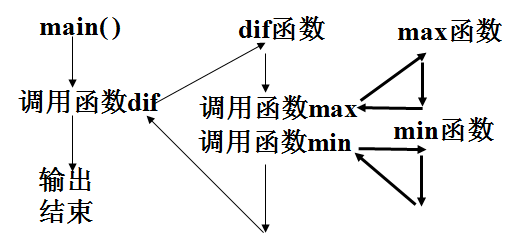例：数的分离
【问题描述】

【输入格式】

【输出格式】

【输入样例】
31859 3
【输出样例】
8
#include<bits/stdc++.h>
using namespace std;
int digit(long long n,int k)
{   int tmp;
for(int i = 1; i <= k; i++)
{   tmp = n % 10;
n = n / 10;
}
return tmp;
}
int main()
{   long long n;
int k;
cin >> n >> k;
cout << digit(n,k) << endl;
return 0;
}

例、统计闰年
【问题描述】

【输入格式】

【输出格式】

【输入样例】
2000 2004
【输出样例】
2
#include<bits/stdc++.h>
using namespace std;
bool rn(int n)
{   if((n % 4 == 0) && (n % 100 != 0) || (n % 400 == 0)) return true;
else return false;
}
int main()
{   int x,y,t = 0;
cin >> x >> y;
for(int i = x; i <= y; i++)
if(rn(i)) t++;
cout << t << endl;
return 0;
}

### 动手练习：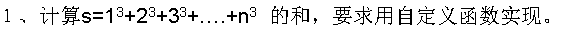２、计算s=1!+2!+3！+….+n!  的和，要求用自定义函数实现。

３、 编写函数，函数功能是：判断输入的字符是否为数字字符。如果是，则输出YES，否则输出NO，要求输入输出均在主函数中完成。

４、编写两个函数，函数功能分别是：求两个整数的最大公约数和最小公倍数，要求输入输出均在主函数中完成。

５、编写函数digit(num, k)，函数功能是：求整数num从右边开始的第k位数字的值，如果num位数不足k位则返回0。要求输入输出均在主函数中完成。

６、编写函数，求一个字符串的长度。在主函数中调用该函数，输出结果。输入字符串（允许包含空格），输出字符串的长度。

７、输入一个字符串，将其中所有的小写字母转换成大写字母。要求用自定义函数实现。

#include<bits/stdc++.h>
using namespace std;
int main()
{  char a;
gets(a);
strupr(a);
cout<<a;
}


#include<bits/stdc++.h>
using namespace std;
int main()
{  char a;
int i,l;
gets(a);
l=strlen(a);
for(i=0;i<l;i++)
if(islower(a[i]))
a[i]=toupper(a[i]);
cout<<a;
}


## 八、内联函数(内置函数）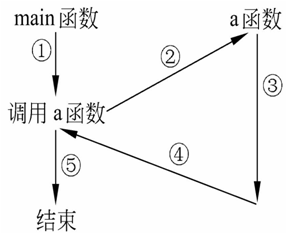C++提供一种提高效率的方法，即在编译时将所调用函数的代码直接嵌入到主调函数中，而不是将流程转出去。这种嵌入到主调函数中的函数称为内置函数(inline function)，又称内嵌函数。在有些书中把它译成内联函数。

#include <iostream>
using namespace std;
inline int max(int,int, int);     //声明函数，左端有inline

int main( )
{  int i=10,j=20,k=30,m;
m=max(i,j,k);
cout<<"max="<<m<<endl;
return 0;
}

inline int max(int a,int b,int c)  //定义max为内置函数
{  if(b>a) a=b;
if(c>a) a=c;
return a;
}


#include <iostream>
using namespace std;
inline int max(int,int, int);
int main( )
{  int i=10,j=20,k=30,m;
if (j>i) i=j;            //语句m=max(i,j,k); 被置换
if(k>i) i=k;
m=i;
cout<<"max="<<m<<endl;
return 0;
}
inline int max(int a,int b,int c)
{  if(b>a) a=b;
if(c>a) a=c;
return a;
}


１、可以在声明函数和定义函数时同时写inline，也可以只在其中一处声明inline，都能按内置函数处理。

２、使用内置函数可以节省运行时间，但却增加了目标程序的长度。因此一般只将规模很小(一般为5个语句以下)而使用频繁的函数声明为内置函数。

３、内置函数中不能包括复杂的控制语句，如循环语句switch语句。

４、对函数作inline声明，只是程序设计者对编译系统提出的一个建议，而不是指令性的。并非一经指定为inline，编译系统就必须这样做。编译系统会根据具体情况决定是否这样做

５、归纳起来，只有那些规模较小而又被频繁调用的简单函数，才适合于声明为inline函数。

## 九、函数重载

### 1、概念

int　　   max1(int a，int b, int c);                         //求3个整数中的最大者

double   max2(double a，double b，double c);  //求3个双精度数中最大者

long  　 max3(long a，long b，long c);             //求3个长整数中的最大者

C++允许用同一函数名定义多个函数，即相同函数名，但是在形参的个数、类型与顺序上有所不同，编译器会根据实参，与形参进行最佳匹配，自动确定应该调用哪个函数， 使一个函数名可以多用，这就是函数的重载。

### 2、举例

#include <iostream>
using namespace std;
int main( )
{  int    max(int a,int b,int c);
double max(double a,double b,double c);
long   max(long a,long b,long c);
int  i1, i2, i3, i;
cin>>i1>>i2>>i3;
i=max(i1,i2,i3);
cout<<"i_max="<<i<<endl;
double d1, d2, d3, d;
cin>>d1>>d2>>d3;
d=max(d1,d2,d3);
cout<<"d_max="<<d<<endl;
long g1,g2,g3,g;
cin>>g1>>g2>>g3;
g=max(g1,g2,g3);
cout<<"g_max="<<g<<endl;
}

int max(int a,int b,int c)            //定义求3个整数中的最大者的函数
{  if(b>a) a=b;
if(c>a) a=c;
return a;
}

double max(double a,double b,double c)//定义求3个双精度数中的最大者的函数
{  if(b>a) a=b;
if(c>a) a=c;
return a;
}

long max(long a,long b,long c)       //定义求3个长整形数中的最大者的函数
{  if(b>a) a=b;
if(c>a) a=c;
return a;
}


185  -76  567 　　　　(输入3个整数)

56.87  90.23  -32.78  (输入3个实数)

67854  -9156  673456 (输入3个长整数)

i_max=567      (输出3个整数的最大值)

d_max=90.23 (输出3个双精度数的最大值)

g_max=673456 (输出3个长整数的最大值)

#include <iostream>
using namespace std;
int main( )
{  int max(int a,int b,int c);          //函数声明
int max(int a,int b);                  //函数声明
int a=8,b=-12,c=27;
//输出3个整数中的最大者
cout<<"max(a,b,c)="<<max(a,b,c)<<endl;
//输出两个整数中的最大者
cout<<"max(a,b)="<<max(a,b)<<endl;
}
//此max函数的作用是求3个整数中的最大者
int max(int a,int b,int c)
{  if(b>a) a=b;
if(c>a) a=c;
return a;
}
//此max函数的作用是求两个整数中的最大者
int max(int a,int b)
{  if(a>b) return a;
else return b;
}


max(a,b,c)=27

max(a,b)=8

### 3、总结

例如：

int    f(int);            //函数返回值为整型

long f(int);            //函数返回值为长整型

void f(int);            //函数无返回值

在函数调用时都是同一形式，如“f(10)”。编译系统无法判别应该调用哪一个函数。重载函数的参数个数、参数类型或参数顺序3者中必须至少有一种不同，函数返回值类型可以相同也可以不同。

在使用重载函数时，同名函数的功能应当相同或相近，不要用同一函数名去实现完全不相干的功能，虽然程序也能运行，但可读性不好，使人莫名其妙。

## 十、实参与形参

### 1、概念

（简称实参）

1、对于形参和实参的说明：

(1) 对于被调用函数中的形参变量，在程序未调用该函数前，并不占内存的存储空间，只有在被调用函数max执行时，形参变量才被分配临时存储空间。函数调用完毕，形参变量的存储空间将被内存收回。

(2)实参可以为常量、变量或表达式、数组元素、数组名、指针、结构体变量、枚举变量、对象、函数名等。如  show2(i)    但要求 i 有确定的值。以便在调用函数时将实参的值赋给形参。

(3)在定义函数时,必须在函数首部指定形参的类型。

(4)实参与形参的类型应相同或赋值兼容。实参和形参都是整型,这是合法的、正确的。如果实参为整型而形参为实型,或者相反,则按不同类型数值的赋值规则进行转换。例如实参a的值为3.5,而形参x为整型,则将3.5转换成整数3。字符型与整型可以互相通用。

(5)实参变量对形参变量的数据传递是“值传递”,单向传递,只由实参传给形参,而不能由形参传回给实参。在调用函数时,编译系统临时给形参分配存储单元。

请注意:实参单元与形参单元是不同的存储单元。

2．形参和实参的结合：

(1) 个数一致：形参有多少个数据项，在调用时实参就必须有多少个数据项

(2) 顺序一致：若要把实参v的值传递给形参w，在调用时v在实参中的顺序必须等于w在形参中的顺序，数据类型也要保持一致。

(3)实参表求值顺序， 自右向左。

#include<bits/stdc++.h>
int f(int a, int b);
int main()
{   int i=2,p;
p=f(i,++i);
printf("%d",p);
}
int f(int a, int b)
{   int c;
if(a>b)  c=1;
else if(a==b)   c=0;
else c=-1;
return(c);
}

#include<bits/stdc++.h>
int f(int a, int b);
int main()
{   int i=2,p;
p=f(i,i++);
printf("%d",p);
}
int f(int a, int b)
{   int c;
if(a>b)  c=1;
else if(a==b)   c=0;
else c=-1;
return(c);
}


3、有默认参数的函数：

如    area(7.5);        //形参得到的值为7.5，而不是6.5

volume(45.6);                        //相当于volume(45.6,12.5)

volume(34.2,10.4)                 //h的值为34.2r的值为10.4

void f1(float aint b=0int cchar d=′a′);     //不正确

void f2(float aint cint b=0, char d=′a′);     //正确

f2(3.5, 5, 3, ′x′)           //形参的值全部从实参得到

f2(3.5, 5, 3)                      //最后一个形参的值取默认值′a′

f2(3.5, 5)                          //最后两个形参的值取默认值，b=0,d=′a′

可以看到，在调用有默认参数的函数时，实参的个数可以与形参的个数不同，实参未给定的，从形参的默认值得到值。利用这一特性，可以使函数的使用更加灵活。例如求2个数或3个数中的最大数,也可以不用重载函数，而改用带有默认参数的函数。

#include <iostream>
using namespace std;
int main( )
{int max(int a, int b, int c=0);           //函数声明,形参c有默认值
int a,b,c;
cin>>a>>b>>c;
cout<<“max(a,b,c)=”<<max(a,b,c)<<endl;    //输出3个数中的最大者
cout<<“max(a,b)=”<<max(a,b)<<endl;        //输出2个数中的最大者
return 0;
}
int max(int a,int b,int c)                 //函数定义
{if(b>a) a=b;
if(c>a) a=c;
return a;
}


14  -56  135

max(a,b,c)=135

max(a,b)=14

(1)如果函数的定义在函数调用之后，则在函数调用之前需要有函数声明，此时必须在函数声明中给出默认值，在函数定义时不允许给默认值。　 如果函数的定义在函数调用之前，则应在函数定义中给出默认值。

(2) 一个函数不能既作为重载函数，又作为有默认参数的函数。因为当调用函数时如果少写一个参数，系统无法判定是利用重载函数还是利用默认参数的函数，出现二义性，系统无法执行。

## 十一、函数调用时参数的传递

#include <bits/stdc++.h>
using namespace std;
void swap(int x, int y); //函数原型

int main()
{  int a=15,  b=18;
swap (a, b);
cout<<"a="<<a<<"   b="<<b<<endl;
}

void swap(int x, int y)
{   int temp;
cout<<"x="<<x<<"   y="<<y<<endl;
temp=x;     x=y;     y=temp;
cout<<"x="<<x<<"   y="<<y<<endl;
}


x=       y=

a=       b=

#include <bits/stdc++.h>
using namespace std;
void swap(int &x, int &y); //函数原型

int main()
{  int a=15,  b=18;
swap (a, b);
cout<<"a="<<a<<"   b="<<b<<endl;
}

void swap(int &x, int &y)     //参数前加&地址符
{   int temp;
cout<<"x="<<x<<"   y="<<y<<endl;
temp=x;     x=y;     y=temp;
cout<<"x="<<x<<"   y="<<y<<endl;
}


x=       y=

a=       b=

1、在函数未被调用时，函数的形参并不占有实际的内存空间，也没有实际的值。

2、只有在函数被调用时才为形参分配存储单元，并将实参与形参结合。

3、实参类型必须与形参相符。

4、函数的参数传递指的就是形参与实参结合的过程。

5、函数进行参数传递，是在栈空间里为形参分配空间，并将计算各个实参的表达式的值，然后一一拷贝给形参的过程。

6、根据函数的参数传递的不同，函数调用可以分为：

函数的值传递调用（传值调用

函数的指针传递调用（传址调用

函数的引用传递调用（引用调用

### 1、传值调用

#include <bits/stdc++.h>
using namespace std;
void swap(int x, int y); //函数原型
int main()
{  int a=15,  b=18;
swap (a, b);
cout<<"a="<<a<<"   b="<<b<<endl;
}
void swap(int x, int y)
{   int t;
cout<<"x="<<x<<"   y="<<y<<endl;
t=x;     x=y;     y=t;
cout<<"x="<<x<<"   y="<<y<<endl;
}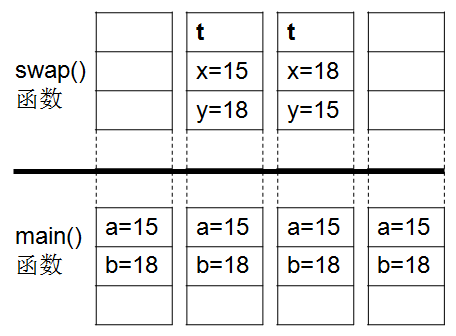1、要传递的参数是一个比较大的自定义的复杂数据类型，如果按值转递方式，需要将这个复杂的数据类型在被调用的函数的栈空间复制一份，这样对时间和空间的开销都比较大。

2传值调用只能通过return语句返回一个值给函数，如果有时需要在被调用函数里改变实参变量的值。则可以使用函数的指针传递调用的方式和引用传递调用来传递参数。这实际就是向主调函数传递多个值

### 二、传址调用

#include <bits/stdc++.h>
using namespace std;
void swap(int *x, int *y); //函数原型
int main()
{  int a=15,  b=18;
swap (&a, &b);
cout<<"a="<<a<<"   b="<<b<<endl;
}
void swap(int *x, int *y)
{   int t;
cout<<"x="<<x<<"   y="<<y<<endl;
cout<<"x="<<*x<<"   y="<<*y<<endl;
t=*x;     *x=*y;     *y=t;
cout<<"x="<<*x<<"   y="<<*y<<endl;
}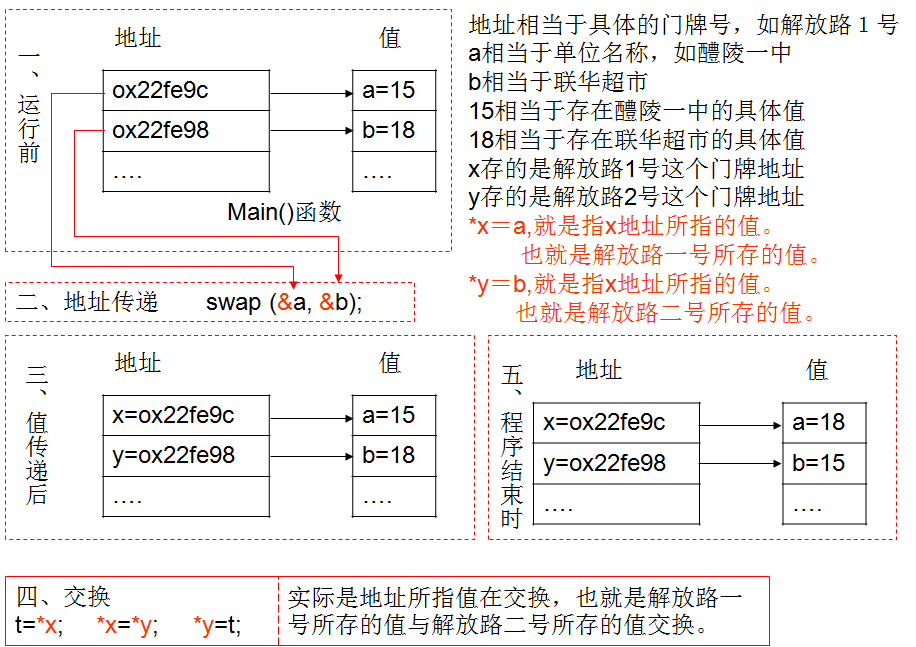### 三、引用调用

引用的声明方法：类型标识符 &引用名=目标变量名；

例：int a; int &x=a;    //定义引用ra,它是变量a的引用，即别名

（1&在此不是求地址运算，而是起标识作用。

（2）类型标识符是指目标变量的类型。

（3）声明引用时，必须同时对其进行初始化。

（4）引用声明完毕后，相当于目标变量名有两个名称，即该目标原名称和引用名，且不能再把该引用名作为其他变量名的别名。　　 x=1; 等价于 a=1;

（5）声明一个引用，不是新定义了一个变量，它只表示该引用名是目标变量名的一个别名，引用本身不占存储单元。故：对引用求地址，就是对目标变量求地址。&x&a相等。

（6）不能建立数组的引用。因为数组是一个由若干个元素所组成的集合，所以无法建立一个数组的别名。

#include <bits/stdc++.h>
using namespace std;
int main()
{  int a;
int &x=a;
cin>>a;
cout<<"a的地址="<<&a<<endl;
cout<<"x的地址="<<&x<<endl;
cout<<"a的值="<<a<<endl;
cout<<"x的值="<<x<<endl;
}

#include <bits/stdc++.h>
using namespace std;
void swap(int &x, int &y); //函数原型
int main()
{  int a=15,  b=18;
swap (a, b);
cout<<"a="<<a<<"   b="<<b<<endl;
}
void swap(int &x, int &y)
{   int temp;
cout<<"x="<<x<<"   y="<<y<<endl;
temp=x;     x=y;     y=temp;
cout<<"x="<<x<<"   y="<<y<<endl;
}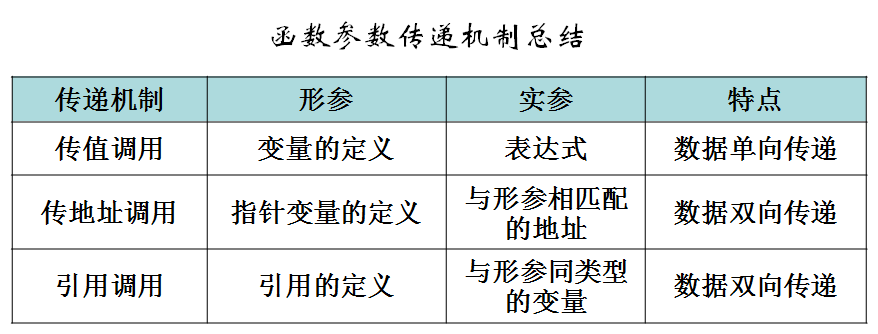## 十二、局部变量与全局变量

C++语言中每个变量和函数都有数据类型数据的存储类别数据作用域三个属性。

具体可分为四种类型： auto自动型        static静态型      register寄存器型             extern外部型

1、作用域之局部变量

• 局部变量：在一个函数内部或复合语句中定义,只在本函数或复合语句内有效的变量称为局部变量，也称为内部变量。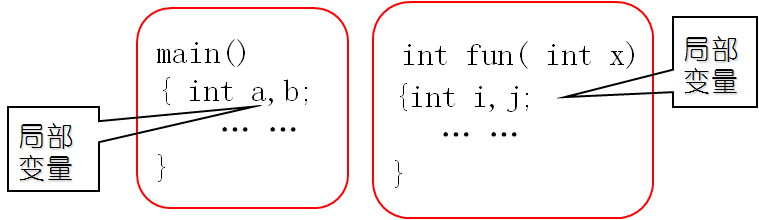• 关于局部变量（内部变量）使用的几点说明：

1、在主函数main中定义的变量a,b也是局部变量，而不因为在主函数中定义而在整个程序中有效，主函数也不能使用其它函数中定义的变量。

2、不同函数中可以使用相同的变量名，但它们占用不同的存储单元，互不干扰；

3、形式参数也是局部变量。其它函数是不能调用该形参的；

4、一个函数中，在复合语句中定义的变量只能在本复合语句中有效,:for(int i=1;i<n;i++)

#include <bits/stdc++.h>
void fund1( void )
{  int nX= 100;              	//局部变量
printf(“nX in fund1=%d\n”, nX);
}
void fund2( void )
{  int nX = 200;	           //局部变量
printf(“nX in fund2=%d\n”, nX);
}
int main( void）
{   int nX =50;	               //局部变量
printf( nX in main=%d\n”, nX);
fund1();
fund2();
｝


nX in main=50

nX in fund1=100

nX in fund2=200

• 不同函数中同名局部变量
#include<bits/stdc++.h>
using namespace std;
void sub()
{ int a,b;
a = 6;  b = 7;
cout<<"sub:a="<<a<<",b="<<b<<endl;
}
int main()
{ int a,b;
a = 3;  b = 4;
cout<<"main:a="<<a<<",b="<<b<<endl;
sub();
cout<<"main:a="<<a<<",b="<<b<<endl;
}


main:a=3,b=4

sub:a=6,b=7

main:a=3,b=4

#include<bits/stdc++.h>
#define  N  5
using namespace std;
int main()
{ int i;
int a[N]={1,2,3,4,5};
for(i=0;i<N/2;i++)
{  int temp;
temp = a[i];
a[i] = a[N-i-1];
a[N-i-1] = temp;
}
for(i=0;i<N;i++)
cout<<a[i];
}


5 4 3 2 1

• 看程序写答案:
#include<bits/stdc++.h>
using namespace std;
int main( )
{  int a=10,b=50;
cout<<"a="<<a<<endl;
{int b=20;
a=a+b;
cout<<"a="<<a<<endl;
cout<<"b="<<b<<endl;
a=15;
}
cout<<"a="<<a<<endl;
cout<<"b="<<b<<endl;
}

#include <stdio.h>
int main( )
{ int x=5;       //缺省为auto自动型变量
printf("x=%d\t",x);
if(x>0)
{  int x=10;  //x为复合语句局部（内部）变量
printf("x=%d\t",x);
}
printf("x=%d\n",x+2);
}


### 2、作用域之全局变量

• 全局变量：在函数之外定义的变量，从定义变量的位置开始到本源文件结束都有效的变量称为全局变量，也称为外部变量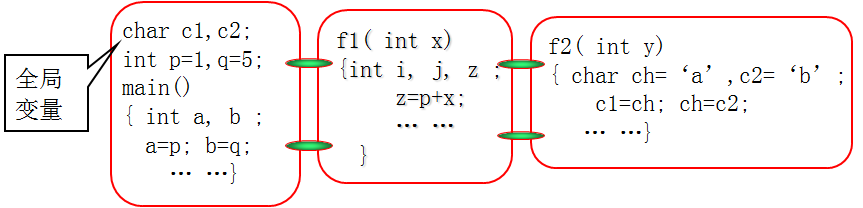• 关于全局变量（外部变量）使用的几点说明

1、若在同一个源文件中，如有全局变量与局部变量同名时，则在局部变量的作用范围内，全局变量不起作用。

2、设置全局变量的作用是: 增加函数间数据联系的渠道。

3、由于同一个文件中的所有函数都能引用全局变量的值，因此如果在一个函数中改变了全局变量的值，就能影响到其它函数，相当于各个函数间有直接的传递通道。一般情况不使用全局变量。

4、它的使用范围: 从定义变量的位置开始到本源程序文件结束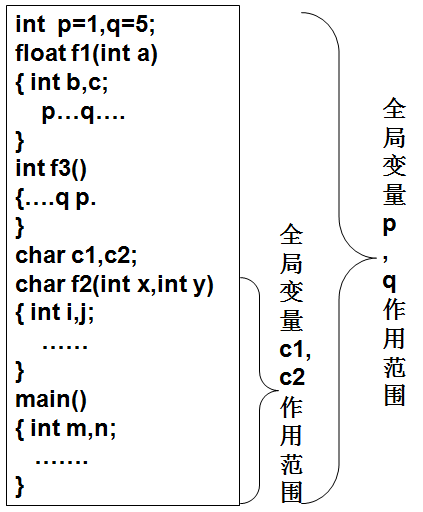• 看程序写答案
#include <iostream>
using namespace std;
void max( );
int s,smax; //定义外部数组和变量
int main()
{ int i;
for(i=0;i<5;i++)
cin>>s[i];
max();
cout<<smax<<endl;
}
void max()
{ int i;
smax=s;
for(i=1;i<5;i++)
if(s[i]>smax)  smax=s[i];
}


#include<bits/stdc++.h>
void fun(int p);
int d=1;
main()
{   int a=3;
fun(a); d+=a++;
printf("%d\n", d);
}
void fun (int p)
{   int d=5;
d+=p++;
printf("%d\n",d);
return;
}

• 全局变量的副作用
#include<bits/stdc++.h>
using namespace std;
int  i,j;
main()
{   void  prt();
for(i=0;i<5;i++)
{  cout<<i<<endl;
prt();
}
}
void prt()
{   for(i=0;i<5;i++)
cout<<"*"<<i;
cout<<endl;
}


### 3、总结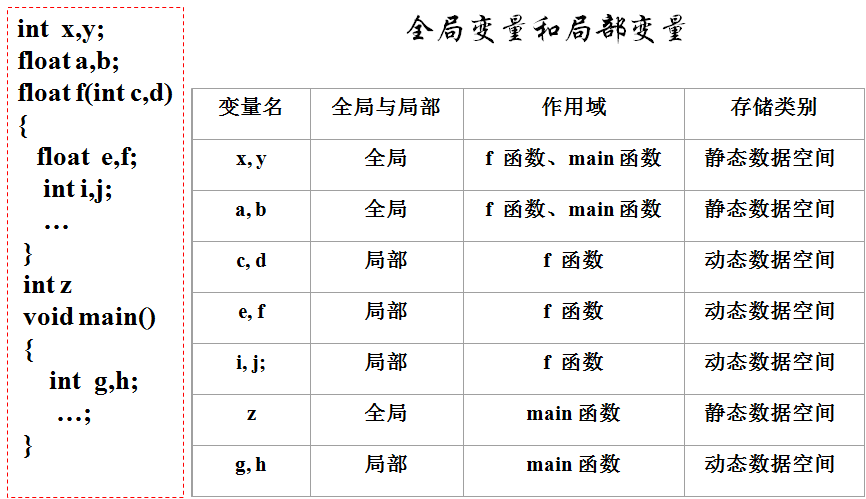## 十三、变量的存储类别

C++语言中每个变量和函数都有数据类型数据的存储类别数据作用域三个属性。

具体可分为四种类型： auto自动型        static静态型      register寄存器型             extern外部型

auto    int   a,b,c;

register   int   i;

static       float   x,y;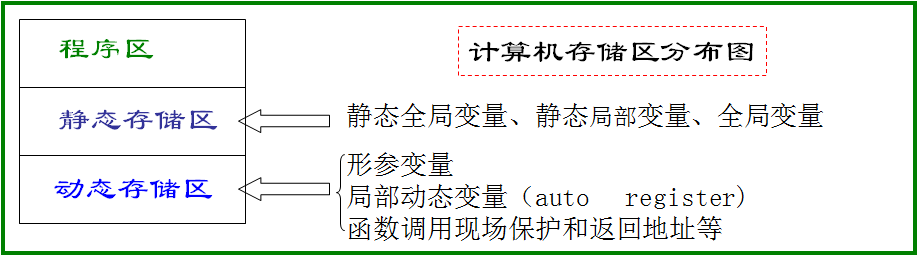生存期：在程序运行期间分配固定的存储空间，不释放

作用域：从程序开始执行到程序结束

初始化：变量值在程序执行中系统只进行一次初始化；

包    括全局变量（外部变量）和static局部变量， static全局变量

生存期：在程序运行期间根据需要临时分配存储空间，离开即释放

作用域：从包含该变量定义的函数开始执行至函数执行结束

初始化：每次函数调用时都进行初始化；

包    括:   auto型局部变量、register型局部变量，函数形参；

### 1、存储类别之自动变量(auto)

我们以前定义的局部变量就是自动变量

#include<bits/stdc++.h>
using namespace std;
int main()
{  int x=1;                  //等价于auto int  x=1;
void  prt(void);
{  int x=3;               //等价于auto int  x=3
prt();
printf(“2nd x=%d\n”,x);
}
printf(“1st x=%d\n”,x);
}
void  prt(void)
{  auto int x=5;             //等价于int  x=1;
printf(“3th  x=%d\n”,x);
}


auto变量包括：

1、函数中定义的局部变量。

2、函数中的形参。

3、在复合语句中定义的局部变量.

而全局变量不能被说明为此类型。

⑴ 内存分配

⑵ 变量的初值

⑶ 生存期

⑷ 作用域

• 看程序写答案
#include<cstdio>
void fun( )
{ int a=0; //局部auto变量
printf("af=%d\n",++a);
}
int main( )
{ int a=10;//局部auto变量
fun( );
printf("a=%d\n",++a);
fun( );  fun( );
}


#include<cstdio>
int n;//全局变量没有赋初值，
//默认初始化为0；
void show( )
{ auto int i=3;//局部变量
n++; //对全局变量操作
i++;
printf("n=%d i=%d\n",n,i);
{ auto int  i=10;
i++;
printf("now i=%d \n",i);
}
printf("then i=%d\n",i);
}
int main( )
{ int i;
auto int n=1;//与全局变量同名
printf("at first n=%d\n",n);
for(i=1 ; i<3 ; i++)
show( );//main中定义的n无效
printf("at last n=%d",n);
}


### 2、存储类别之寄存器变量(register)

• 寄存器（register）变量说明:

（1）只有auto变量与形式参数可以作为register变量；

（2register变量它与auto类变量的区别在于： register变量的值存放在寄存器中而不是在内存中。寄存器是CPU芯片内部的存储器，访问速度极快。常把一些运行速度有较高要求，需要频繁引用的变量定义为register型；

（3）计算机中寄存器的数量是有限的，而且寄存器的数据长度也是有限的。因此register变量不能定义太多，也不能是数据类型太大的变量(longfloatdouble)

（4）不能对寄存器变量使用取地址运算符&

（5register变量定义通常是不必要的，优化的编译系统能够识别使用频繁的变量，并将其放到寄存器中，而程序员指定的register型变量可能无效。

• 静态变量（static）说明：

（1）内存分配：static变量存放在内存的静态存储区，在整个程序运行期间占用固定的内存单元，程序结束释放该空间。

（2）静态变量的初值：系统在编译时为static变量分配空间并赋初值，对未赋值的局部static数值型变量，系统自动给它赋值为0；对未赋值的局部static字符型变量，自动赋值为空字符。因为在编译时赋初值，所以只能赋一次初值且只能在定义时进行。

（3）生存期：由于变量占用的存储单元不消失，再次调用static局部变量时，static局部变量的值为上次调用结束时的值。

（4）作用域：static局部变量的生存期是整个程序运行期间，但作用域仍然是定义该变量的函数体（或复合语句）内部。  即：静态局部变量在函数调用结束后仍然存在，但其他函数不能引用它。

（5）作用域： static修饰全局变量，作用域是从定义处开始到本源文件结束,将使全局变量作用域局限于本文件内。

（6）可以修饰局部变量和全局变量。

#include<bits/stdc++.h>
void f()
{ static int a;
int x=1;
a++;  x++;
printf("a=%d x=%d ",a,x);
}
int  main()
{ int  i;
for(i=0;i<3;i++)  f( );
}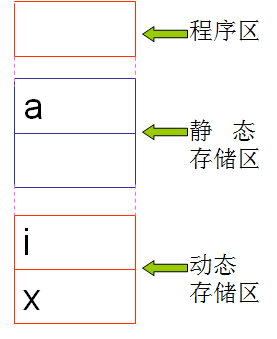1、定义的静态变量在编译时，系统分赋初值0或空字符。

2、变量占用的存储单元不消失，再次调用static局部变量时，static局部变量的值为上次调用结束时的值。

3、静态局部变量只在本函数或本复合语句中有效，在函数调用结束后仍然存在，但其他函数不能引用它。

06-071993
04-255489
10-02733
04-29997
07-20487
08-08812
03-131684
08-16352
09-13229
04-151066
12-235837
10-15228
05-201302
10-30675
09-18179
03-31887
04-138万+
01-02936

### “相关推荐”对你有帮助么？

•非常没帮助
•没帮助
•一般
•有帮助
•非常有帮助

©️2022 CSDN 皮肤主题：大白 设计师：CSDN官方博客被折叠的  条评论 为什么被折叠?到【灌水乐园】发言¥2 ¥4 ¥6 ¥10 ¥20余额支付 (余额：-- )扫码支付获取中扫码支付点击重新获取扫码支付1.余额是钱包充值的虚拟货币，按照1:1的比例进行支付金额的抵扣。
2.余额无法直接购买下载，可以购买VIP、C币套餐、付费专栏及课程。余额充值